Test: Functions

# Test: Functions - Commerce

Test Description

## 15 Questions MCQ Test - Test: Functions

Test: Functions for Commerce 2023 is part of Commerce preparation. The Test: Functions questions and answers have been prepared according to the Commerce exam syllabus.The Test: Functions MCQs are made for Commerce 2023 Exam. Find important definitions, questions, notes, meanings, examples, exercises, MCQs and online tests for Test: Functions below.
Solutions of Test: Functions questions in English are available as part of our course for Commerce & Test: Functions solutions in Hindi for Commerce course. Download more important topics, notes, lectures and mock test series for Commerce Exam by signing up for free. Attempt Test: Functions | 15 questions in 15 minutes | Mock test for Commerce preparation | Free important questions MCQ to study for Commerce Exam | Download free PDF with solutions
 1 Crore+ students have signed up on EduRev. Have you?
Test: Functions - Question 1

### Number of subsets of a set of order three is

Test: Functions - Question 2

### If X = {1, 2, 3, 4}, Y = {1, 2, 3,…., 20}, and f: X → Y be the correspondence which assigns each element in X the value equal to its square, then the domain, co-domain and range of f is

Detailed Solution for Test: Functions - Question 2

The correct option is B

Since the function is defined from

XY.So the domain will be the set X which is {1,2,3,4}. Codomain is  what may possibly come out of a function. So codomain is the whole set Y which is {1,2,3,...,20}. Range is The range is the set of possible output values which will be the values in the set of Y which have a pre-image. That is, the value of squares. So the range is {1,4,,9,16}.

Test: Functions - Question 3

### Which of the following is not true?

Detailed Solution for Test: Functions - Question 3

A relation f from a set A to a set B is said to be function if every element of set A has one and only one image in set B. In other words, a function f is a relation such that no two pairs in the relation has the same first element. The notation f : X →Y means that f is a function from X to Y.

Test: Functions - Question 4

The domain of the function f(x)=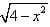is all real x such that

Detailed Solution for Test: Functions - Question 4

4 - x2 = 0
=> (2+x)(2-x) = 0
x = +-2
Now select a test point, let it be x = 0. Then y = (4 - (0)2)1/2 = 2, so the function is defined on [-2,2]
Thus, the graph of y = (4 - x2)1/2 is a semicircle with radius 2 and domain is [-2,2]

Test: Functions - Question 5

Let X = {1, 2, 3, 4}, Y = {1, 2, …, 10} and f : X –> Y be defined by f(x) = 2x + 1, x ∈ X. Then the range of f is

Detailed Solution for Test: Functions - Question 5

X = {1, 2, 3, 4}
Y = {1, 2, …, 10}
f: X→ Y be defined by
f(x) = 2x + 1
f (1) = 3
f (2) = 5
f (3) = 7
f (4) = 9
Range(f) = {3, 5, 7, 9}

Test: Functions - Question 6

If f(x) = x2 – x + 1; g(x) = 7x – 3, be two real functions then (f + g)(3) is

Detailed Solution for Test: Functions - Question 6

f(x) = x2 – x + 1; g(x) = 7x – 3
(f+g)(x) = (x2 - x + 1 + 7x - 3)
=(x2 - x + 7x + 1 - 3)
= x2 + 6x - 2
(f+g)(3) = x2 + 6x - 2
= (3)2 + 6(3) - 2
= 9 + 18 - 2
= 25

Test: Functions - Question 7

Which of the following relations is not a function?

Detailed Solution for Test: Functions - Question 7

A function is a relation in which no two ordered pairs have the same first element. A function associates each element in its domain with one and only one element in its range. R={(1,2), (1,4)(3,1),(5,1)} is not  a function because all the first elements are not different.

Test: Functions - Question 8

The following arrow diagram of the function f : A → A be defined as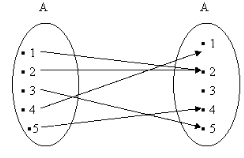Test: Functions - Question 9

If A = {1,2,3}, B = {a,b,c}, which of the following is a function?

Detailed Solution for Test: Functions - Question 9

Option A has value b & c for 2.
Option C has value b & c for 1.
Option D has value a & c for 1.

Test: Functions - Question 10

Which of the relations is not a function?

Detailed Solution for Test: Functions - Question 10

Example: y = x2
If x = 2, y = 4.
If x = -2, y = 4. So there you have a many-to-one relation and it is DEFINITELY a function.
Now, a one-to-many relation is NOT a function.

Test: Functions - Question 11

The domain and range of the function f: R → R defined by: f = {(x+1, x+5): x ∈ {0, 1, 2, 3, 4, 5}}

Detailed Solution for Test: Functions - Question 11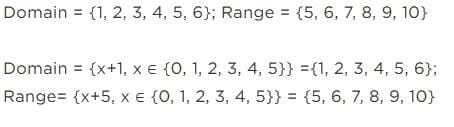Test: Functions - Question 12

Identify the domain and range of the function f(x), defined in N, as below: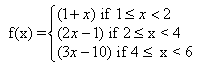Detailed Solution for Test: Functions - Question 12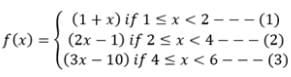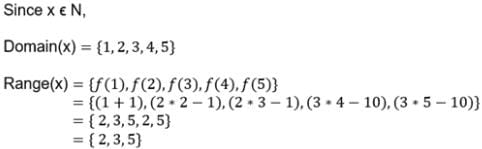Test: Functions - Question 13

If A = {2, 4, 6, 8} and B = {2, 3, 5, 7, 9}. Which of the following is a function from A to B?

Test: Functions - Question 14

Find the domain of the function f(x) = x |x|

Detailed Solution for Test: Functions - Question 14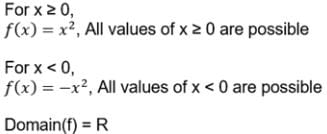Test: Functions - Question 15

Find the domain of the function f(x) = 3x/2x - 8

Detailed Solution for Test: Functions - Question 15

f(x) = 3x/2x-8
denominator can't be zero so
2x - 8 not equal to 0
x should not equal to 4
we have domain R - {4}(Scan QR code)